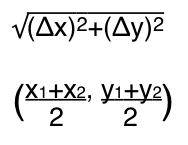# PDF Formula Sheet: Geometry–Coordinate Geometry, Shapes, and SolidsThe distance formula & the midpoint formula

Looking for a shapes/solids or coordinate geometry formula PDF? You are in the right area, alright. Coordinate your studies with this high volume of formulas.

Three puns of that caliber in row and I’ve found the midpoint of self-loathing.

Geeze, I can’t stop. I’m on a downward…slope.

# Formula Sheet: Geometry

Here’s a list of common expressions and equations used in coordinate geometry and the geometry of shapes and solids, including the distance formula and the midpoint formula.Geometry Formula PDF

# Memorize the Geometry Formula Sheet with a Free Game

Our online memorization tool, Study Putty, has a game for committing these geometry formulas to memory. You can study coordinate geometry formulas and the formulas for shapes and solids separately or together. We make it easy to do a SAT Math review or some ASVAB study if you need to brush up on your forgotten formulas with a few practice rounds.What do you need to study on Study Putty? Tell us here!

Bookmark the permalink.## About Bret Norwood

Bret Norwood is a staff blogger for Learning Laboratory in addition to other roles, including UI design and content development for Study Putty, our free memorization tool for chemistry and many other course topics. He is also a published writer of literary fiction--see BretNorwood.com